March 25, 2021

## Outline

1. Confounding
• sources of bias
• direction of bias
2. Solutions to Confounding

## Example:

Recent mass shooting in Boulder, CO has renewed calls in the United States to impose gun control legislation.

## Correlation: Guns and Gun Deaths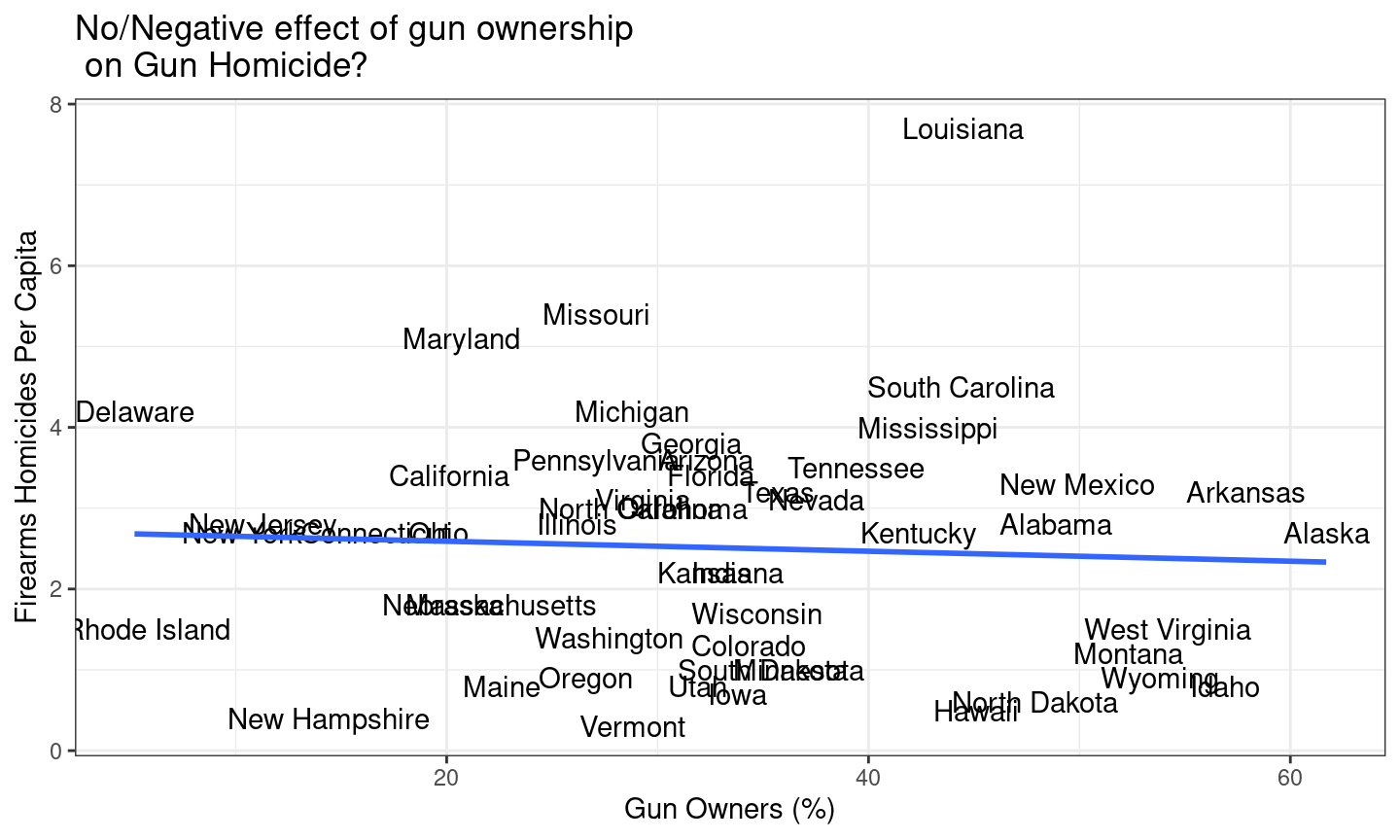POLL

## Confounding?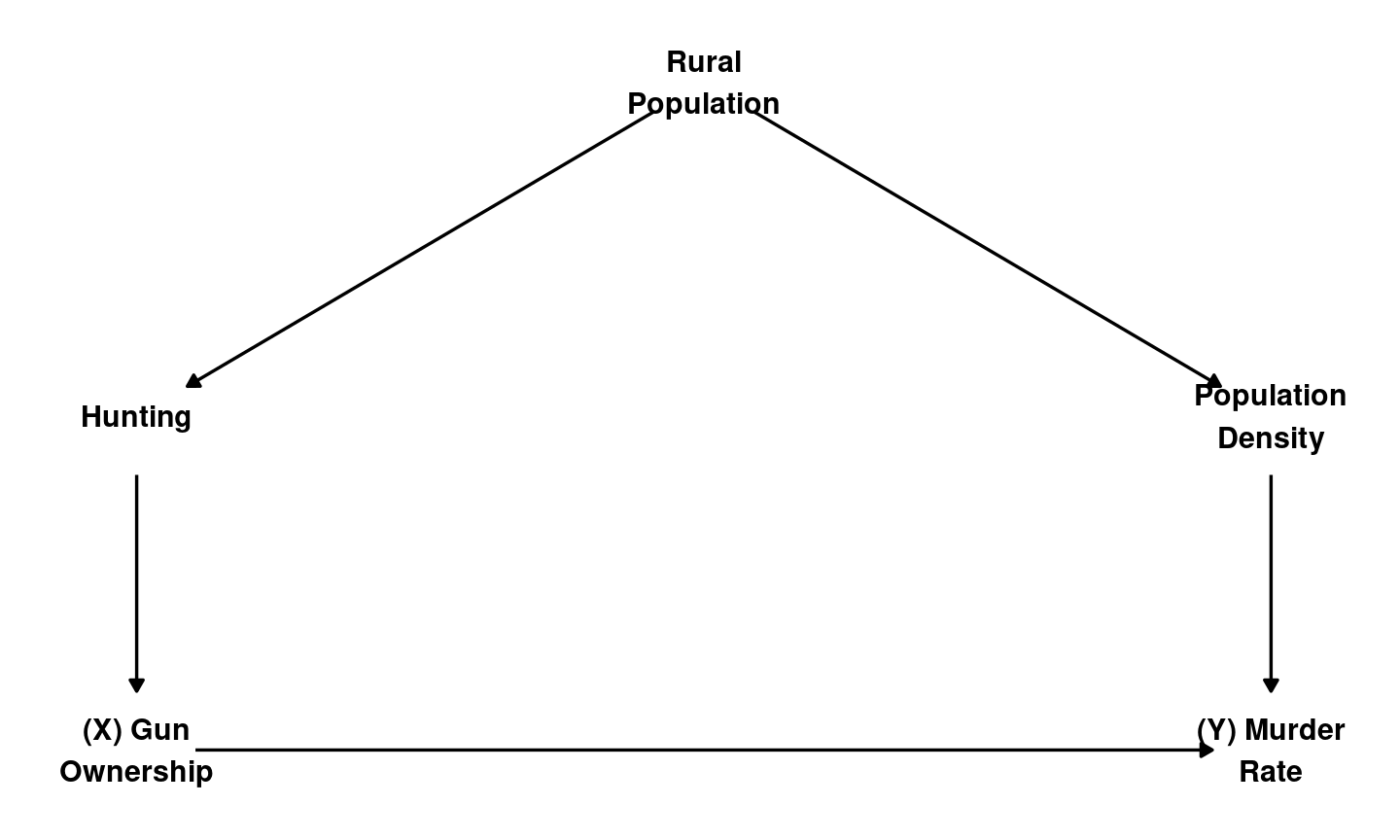## Confounding:

Confounding occurs when these other differences between cases (third variables, e.g. $$W$$) causally affect $$X$$ and $$Y$$.

In a causal graph, there is confounding of correlation of $$X$$ and $$Y$$ if…

1. some variable $$W$$ has causal paths toward $$X$$ and $$Y$$
2. (equivalently) there is backdoor path or non-causal path from $$X$$ to $$Y$$

## Confounding:

Third Variable? Key Attribute Confounding?
Antecedent Variables
($$W$$)
Yes $$W \to X$$ If only causal path from $$W$$ to $$Y$$ contains $$X$$: No
If a causal path from $$W$$ to $$Y$$ excludes $$X$$: Yes
Intervening Variables
($$M$$)
Yes $$X \to M \to Y$$ No
Reverse Causality No $$Y \to X$$ Yes

## Upward or Downward bias?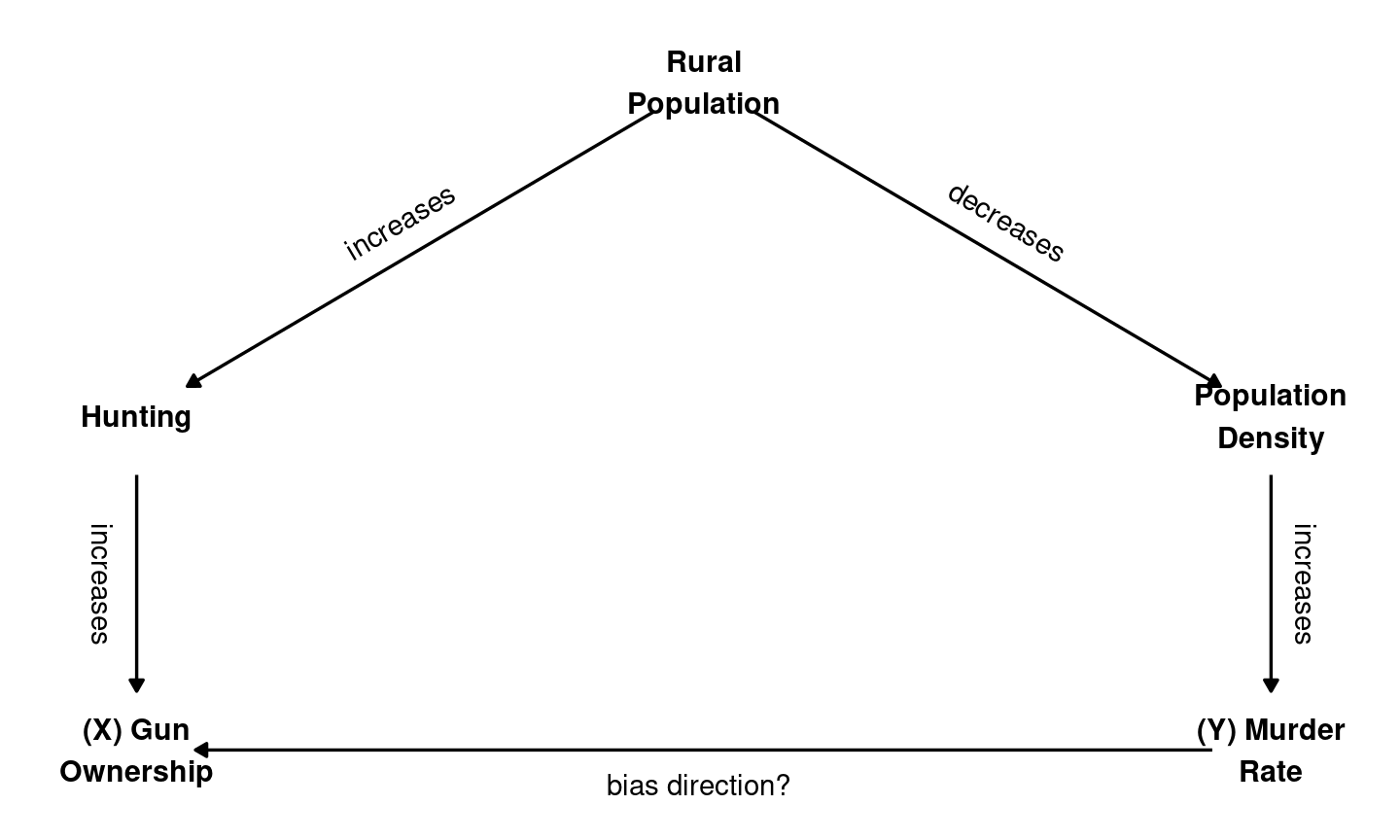## Confounding: Direction of Bias

Product of signs on causal path from $$W \to X$$ and $$W \to Y$$ gives us direction of bias created by confounding

$$W \xrightarrow{+} X$$ $$W \xrightarrow{-} X$$
$$W \xrightarrow{+} Y$$ $$Correlation(X,Y)$$
Biased (+)
$$Correlation(X,Y)$$
Biased (-)
$$W \xrightarrow{-} Y$$ $$Correlation(X,Y)$$
Biased (-)
$$Correlation(X,Y)$$
Biased (+)

## Downward bias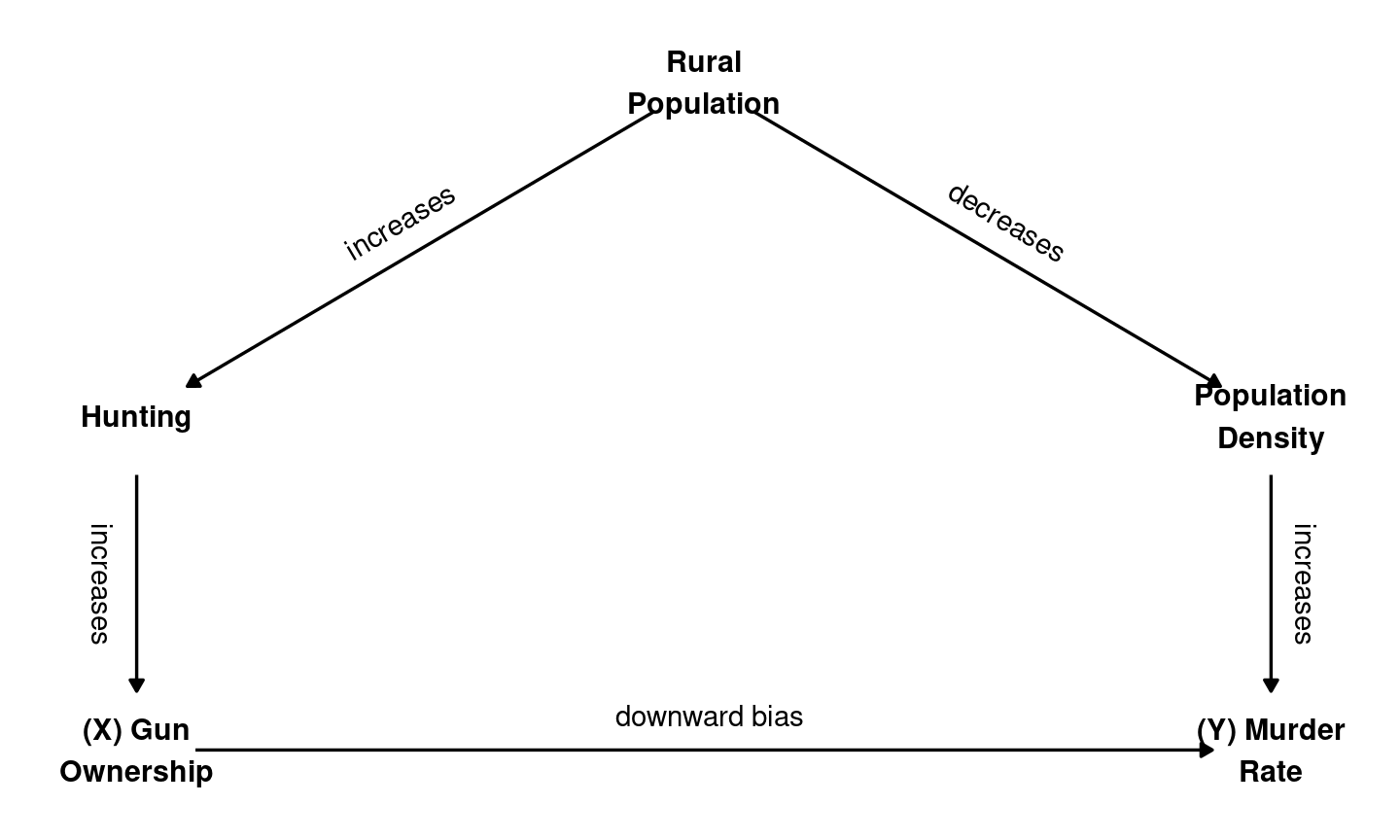## Confounding: Direction of Bias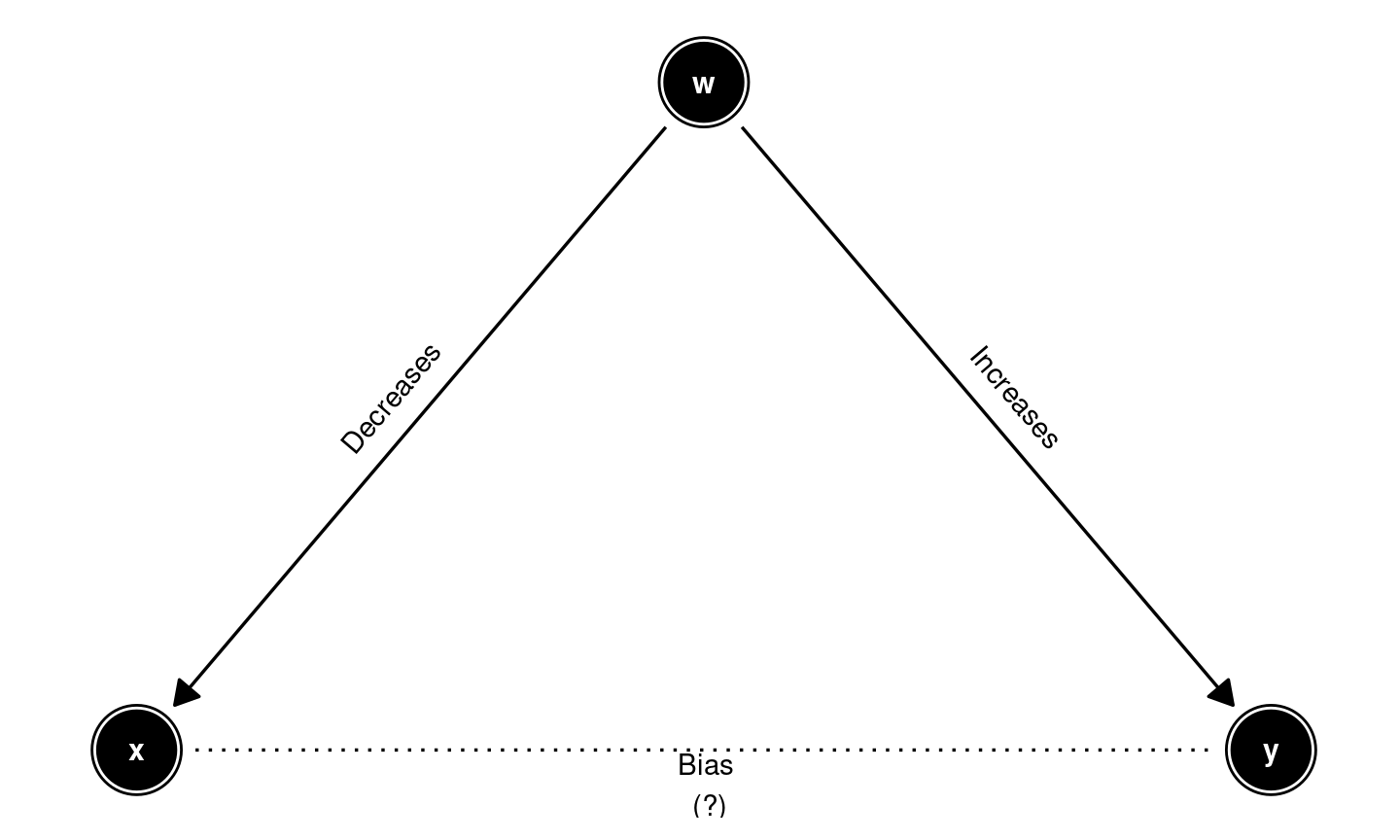## Confounding: Direction of Bias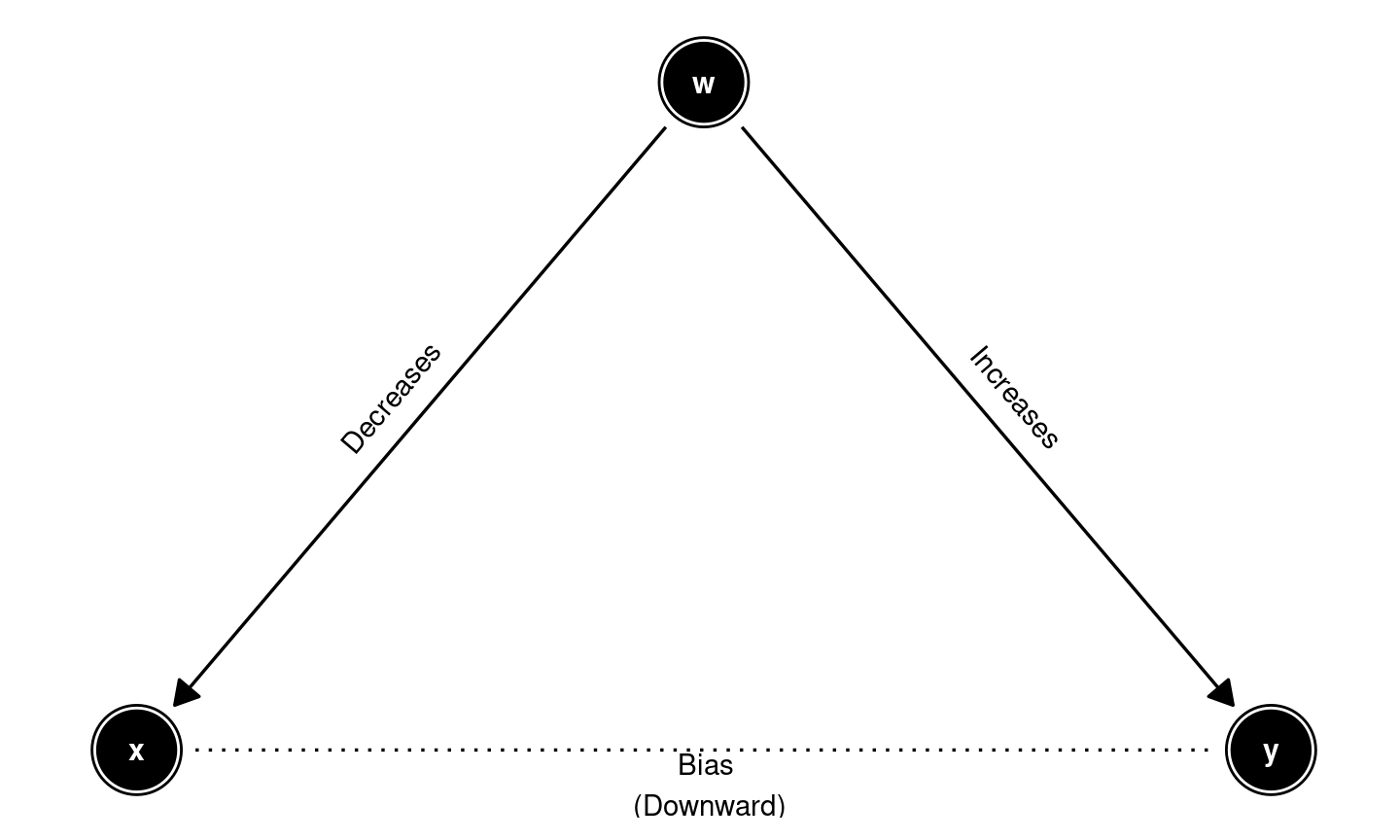## Confounding: Direction of Bias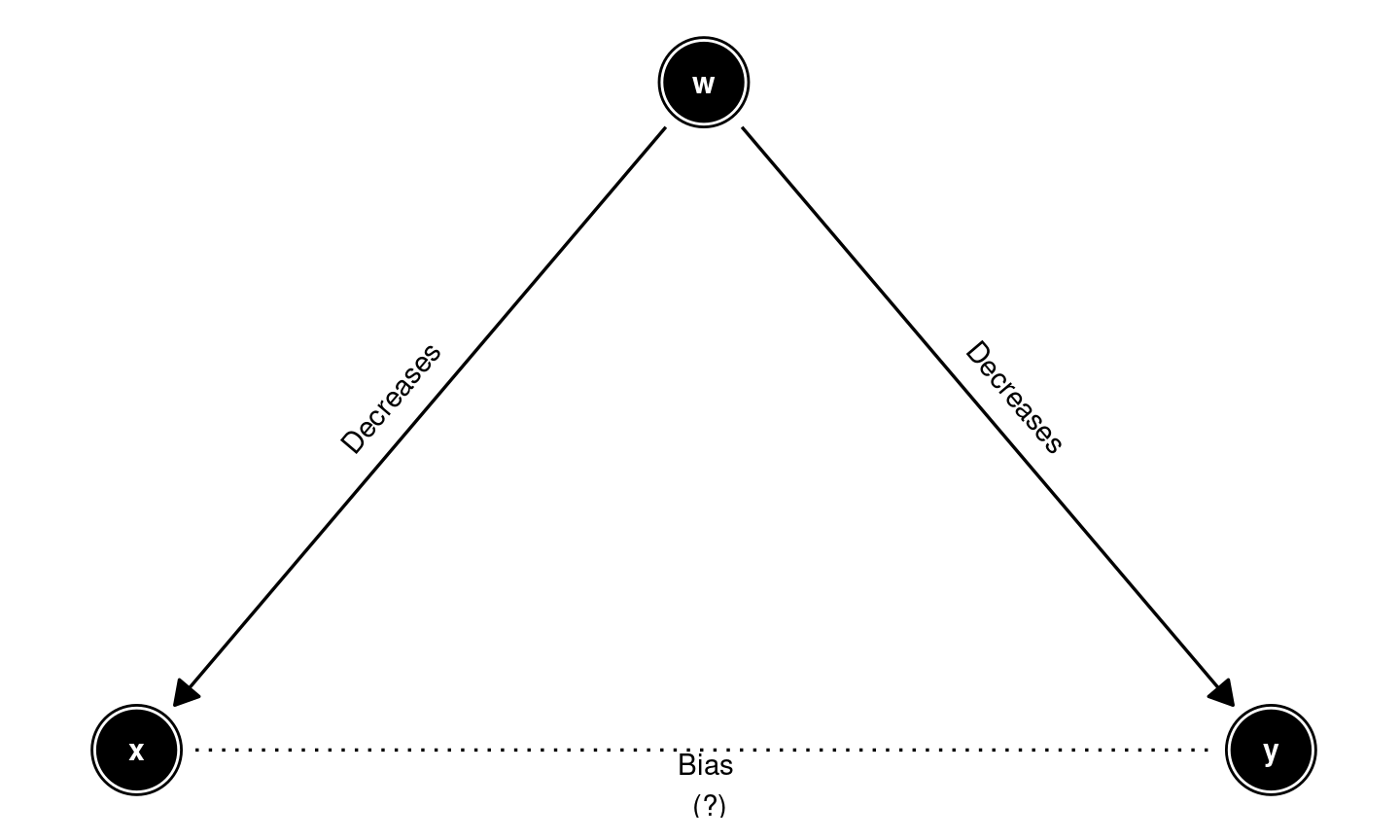## Confounding: Direction of Bias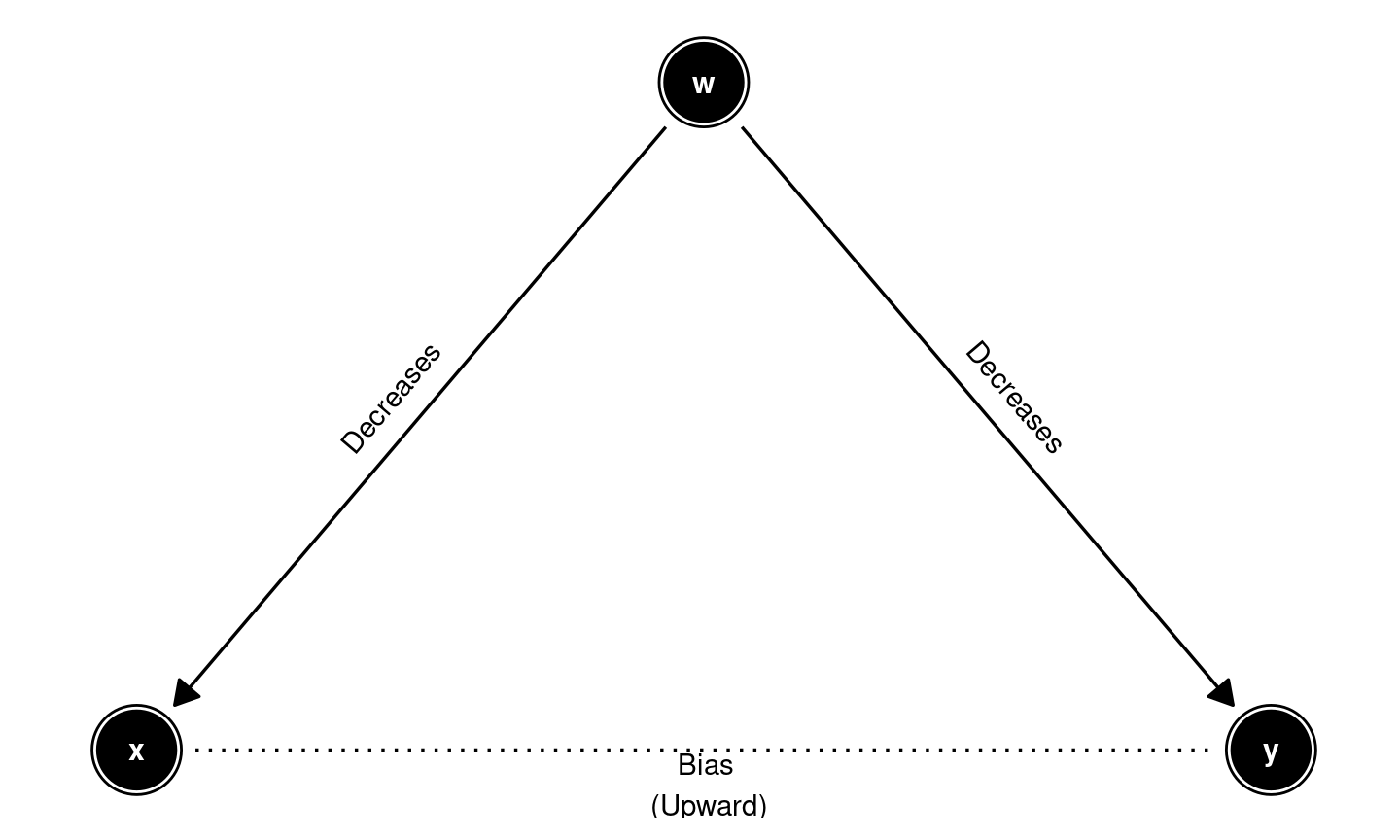## Confounding: Direction of Bias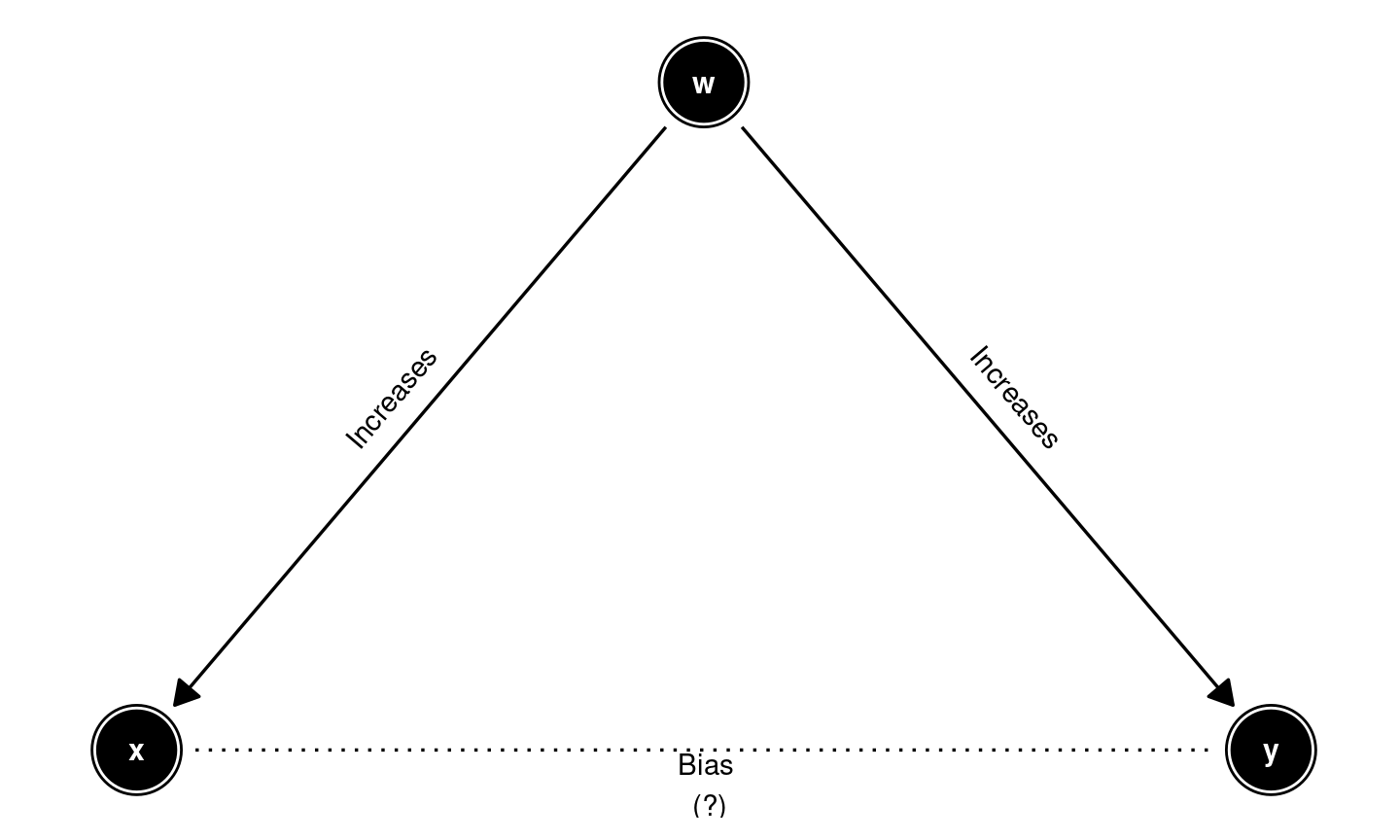## Confounding: Direction of Bias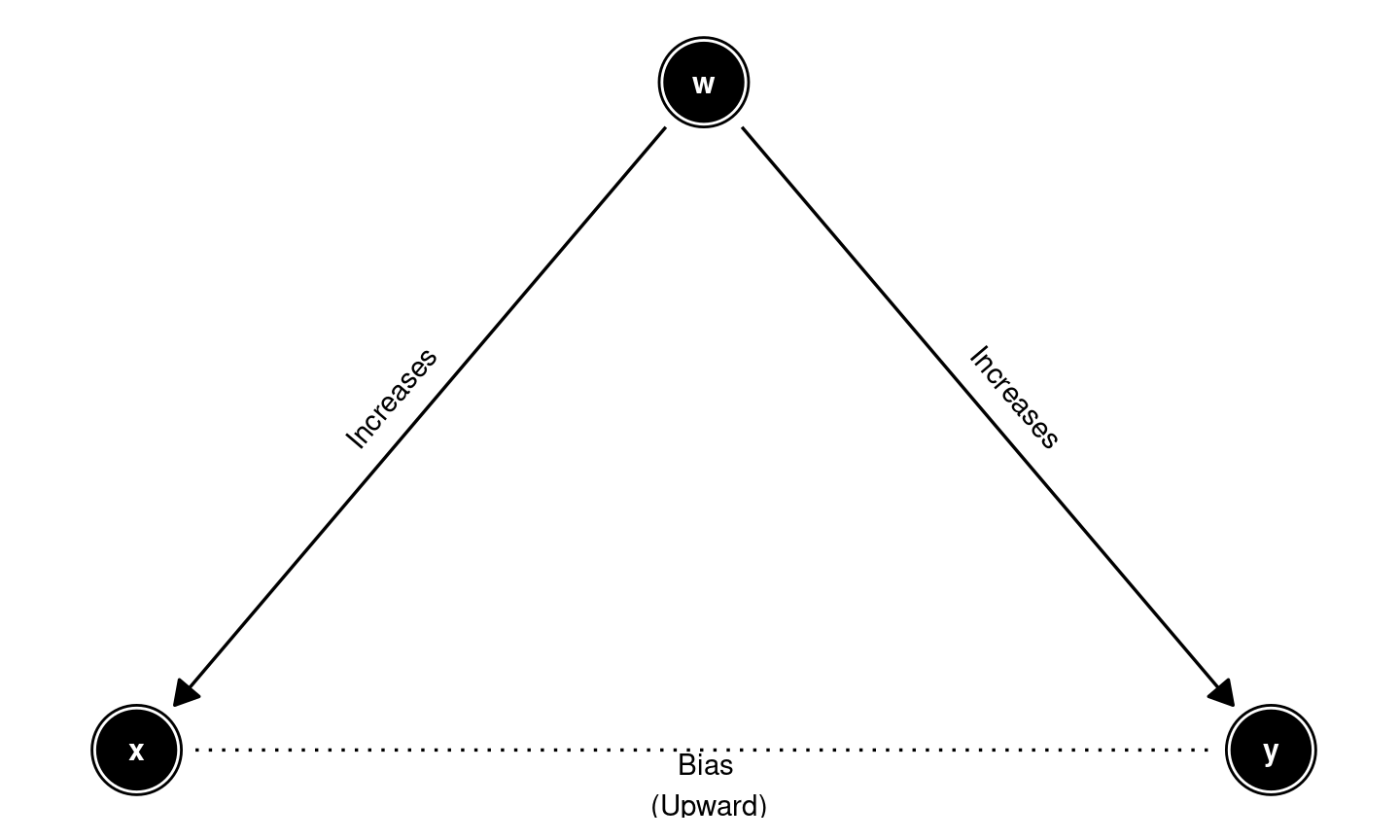## Confounding: Direction of Bias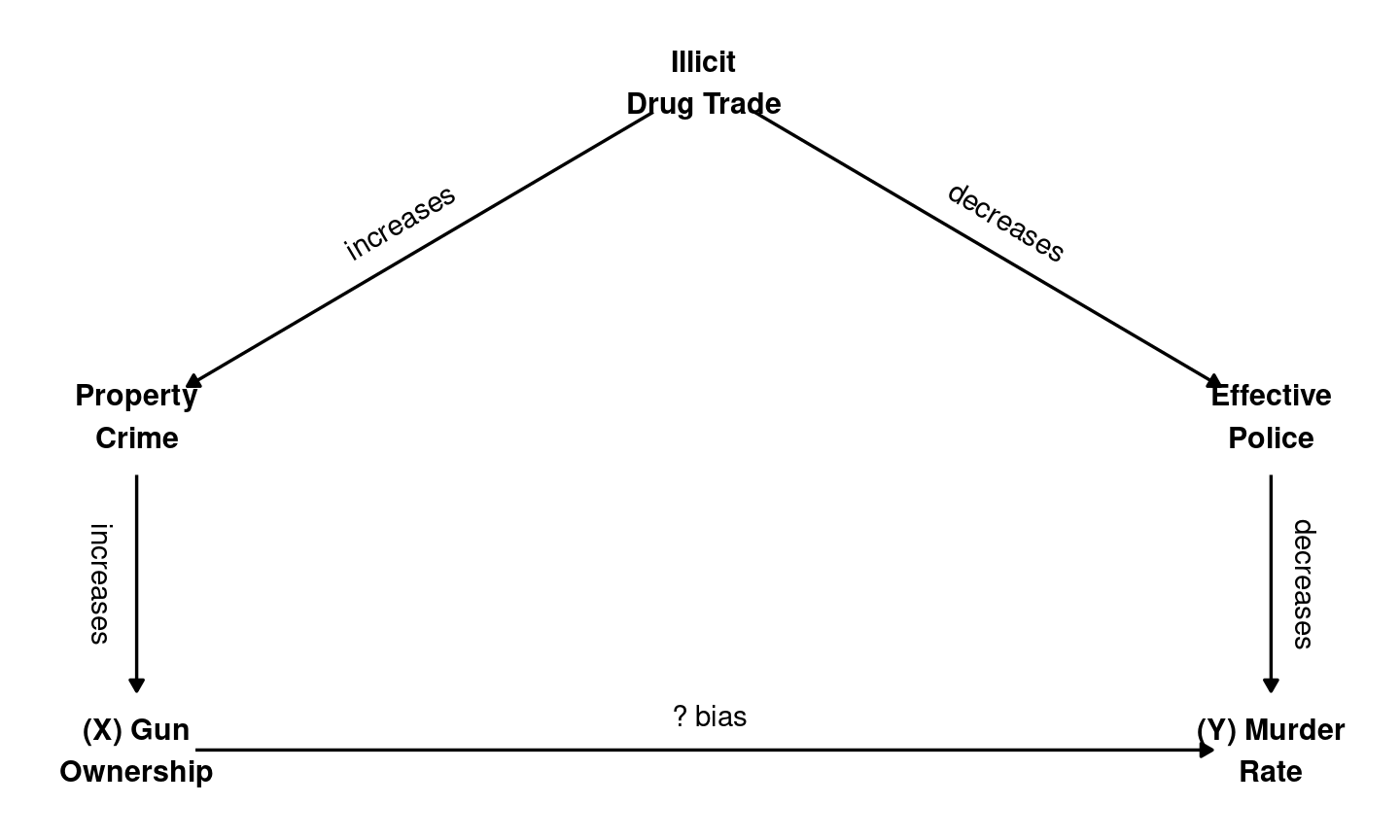## Confounding: Direction of Bias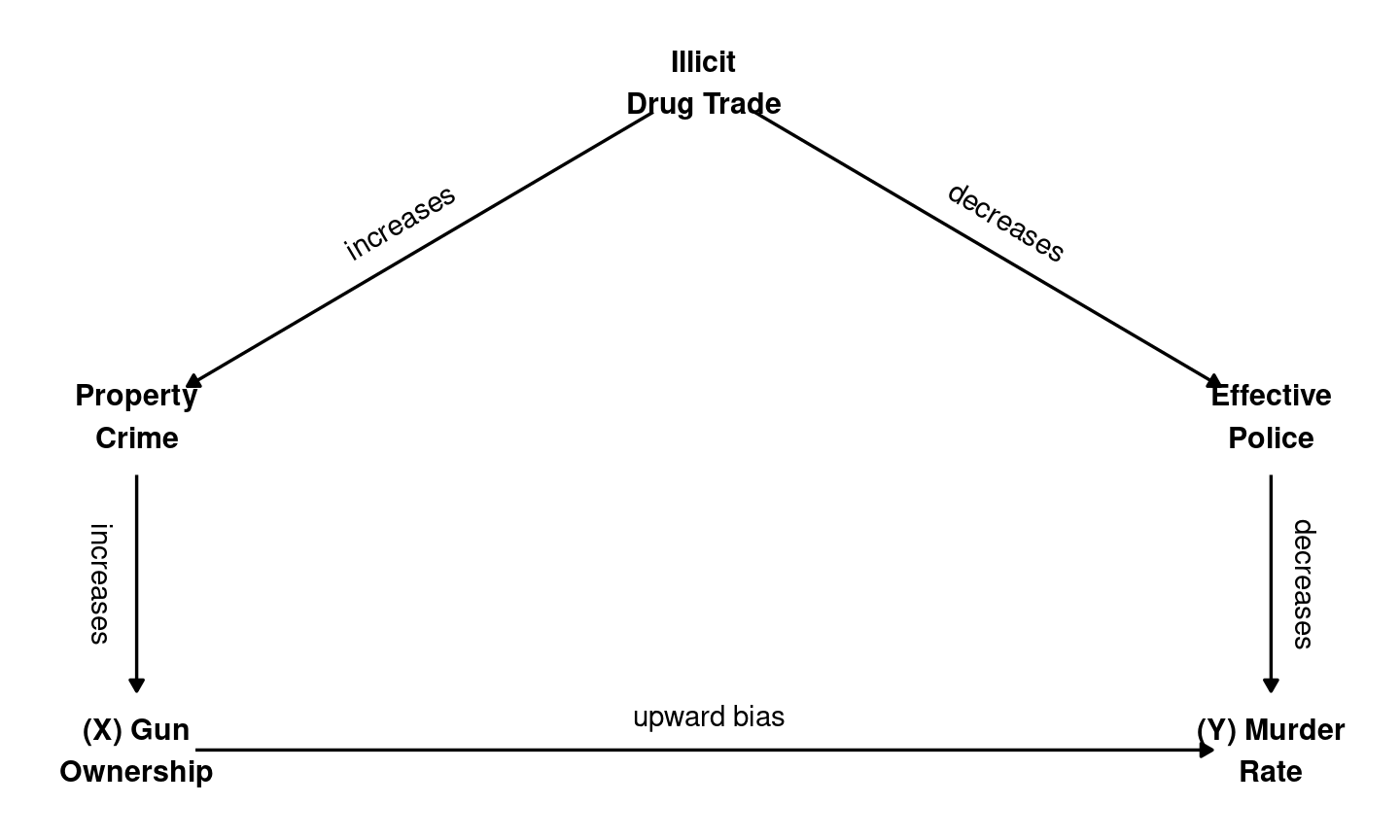## Fixing Confounding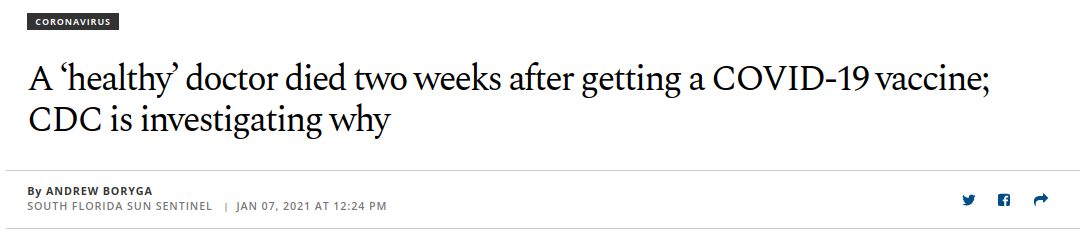POLL

## Pandemic Misinformation

Story above not false, but misleading:

• most widely shared story about the vaccine this year
• $$.0018\%$$ of US vaccine recipients have died
• apprx. 8000 people die in the US die each day for other reasons.

## Pandemic Misinformation

What can be done to limit the negative effects of pandemic misinformation?

• Does thinking about the accuracy of information make people less likely to share misinformation?

## Pandemic Misinformation

What if we survey Facebook users:

• look at previous Facebook history to see if they shared vaccine misinformation
• ask them if they assess the accuracy of information before sharing links

Does a negative correlation imply causation?

• What could we do to avoid confounding?

## Pandemic Misinformation

Pennycook, et al (2020) run this experiment:

• Show people pandemic-related stories that have been independently evaluated as false or true
• “If you were to see the above on social media, how likely would you be to share it?”
• Randomly assign some to assess the accuracy of non-pandemic news before they look at pandemic news.
• People “nudged” to think about accuracy 3.9 ppt more likely to share true rather than false stories

## Experiments

FPCI: We cannot know the causal effect of $$X$$ on $$Y$$ for a specific case.

Correlation of $$X$$ and $$Y$$ for different cases may suffer from confounding

### Experiments are a solution

Allow us to treat correlation as an estimate (an inference about) the average causal effect of $$X$$ on $$Y$$.

• We can’t know the causal effect for individual cases, but can get the average causal effect across all cases

## Experiments

Experiments give us unbiased (no confounding) relationship between $$X$$ and $$Y$$, with assumptions:

1. Random Assignment to “Treatment” and “Control”
2. Exclusion Restriction (only one thing is changing: $$X$$)

Technically, there are more assumptions, but not important for this class

## Experiments

### Randomization solves Confounding

• Randomization balances cases with same potential outcomes in treatment and control
• Randomization balances cases with similar values of confounding variable $$W$$ in treatment and control (breaks the link $$W \to X$$)

Cases are about the same on average: - cases in control are observable “counterfactuals” for cases in treatment - EXACTLY like with random sampling (to the board)

## Experiments

### Exclusion Restriction means we don’t add confounding

If in the COVID misinformation experiment, “Treatment” group …

• Asked to assess the accuracy of information ($$X$$)
• Told that their social media shares were tracked by the government ($$Z$$)

Two things are different between treatment and control group;

• we don’t know which one does the work

## Experiments

Experiments are a solution to confounding/FPCI

• We can’t always use them
• We make trade-offs by using experiments
• What other options are there?